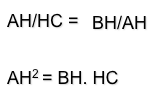# Metric geometry : Theorems height and legTogether with Power Concepts, triangle geometry solves obtaining proportional means through theorems called the height and cateto.

Before stating these theorems and deduce, recall some basic concepts of proportionality to understand what it is that we can solve with constructs derived from these geometric models.

### Fourth proportional

Given the mathematical relationship x/a =b/c call fourth proportional to the value of x, namely

x=a*b/c

### Third proportional

Given the mathematical relationship x/a = a/b called third proportional to the value of x, namely

x=a*a/b

### Proportional Media

Given the mathematical relationship x/a=b/x call mean proportional to the value of x, namely

x = square root of a * b$x=sqrt[a*b]$

In the three cases defined, the ratio can be from models based on similarity and therefore obtained by applying the relationships Thales theorem.

## Triangle geometry

We can obtain a triangle hypotenuse using as a diameter of a circle, and as a point opposite corner of the same, and which defines a arc able 90 grados diameter on said.

If we get the height h the right-angled triangle from (vertex A) and determine its intersection H with the hypotenuse (walk-up) we can determine three triangles similar rectangle:

• ABC
• HAC
• HBA

Thales Applying these three triangles we obtain the following relations:

### Theorem catheterThe leg of a right triangle is the mean proportional between the hypotenuse and the projection of that leg on the hypotenuse.

l*l=m*n

### Height theoremThe height of a right triangle's hypotenuse measure mean proportional between the two segments that divides.

l*l=m*n

Metric geometry